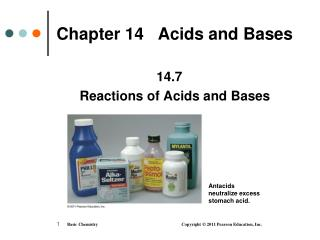DownloadDownload PresentationChapter 14 Acids and Bases

# Chapter 14 Acids and Bases

Télécharger la présentation## Chapter 14 Acids and Bases

- - - - - - - - - - - - - - - - - - - - - - - - - - - E N D - - - - - - - - - - - - - - - - - - - - - - - - - - -
##### Presentation Transcript

1. Antacids neutralize excess stomach acid. Chapter 14 Acids and Bases 14.7 Reactions of Acids and Bases

2. Acids and Metals Acids react with metals • such as K, Na, Ca, Mg, Al, Zn, Fe, and Sn • to produce hydrogen gas and the salt of the metal Chemical equations 2K(s) + 2HCl(aq) 2KCl(aq) + H2(g) Zn(s) + 2HCl(aq) ZnCl2(aq) + H2(g) Net ionic equations 2K(s) + 2H+(aq) 2K+(aq) + H2(g) Zn(s) + 2H+(aq) Zn2+(aq) + H2(g)

3. Acids and Carbonates Acids react with carbonates and hydrogen carbonates to produce carbon dioxide gas, a salt, and water. 2HCl(aq) + CaCO3(s) CO2(g) + CaCl2(aq) + H2O(l) HCl(aq) + NaHCO3(s) CO2(g) + NaCl(aq) + H2O(l)

4. Learning Check Write the products of each of the following reactions as the complete equation and the net ionic equation: A. Zn(s) + 2 HCl(aq) B. MgCO3 (s) + 2HCl(aq)

5. Solution A. Zn(s) + 2 HCl(aq) ZnCl2(aq)+ H2(g) Zn(s) + 2H+(aq)Zn2+(aq)+ H2(g) B. MgCO3(s) + 2HCl(aq) MgCl2(aq) + CO2(g) + H2O(l) MgCO3(s) + 2H+(aq)Mg2+(aq)+ CO2(g) + H2O(l)

6. Neutralization Reactions Neutralization is the reaction of • an acid such as HCl and a base such as NaOH HCl(aq) + H2O(l) H3O+(aq) + Cl−(aq) NaOH(aq) Na+(aq) + OH−(aq) • the H3O+ from the acid and the OH− from the base to form water H3O+(aq) + OH−(aq) 2H2O(l)

8. Neutralization Equations • In the chemical and net ionic equations for neutralization, an acid and a base produce a salt and water. acid base saltwater HCl(aq) + NaOH(aq) NaCl(aq) + H2O(l) H+(aq) + OH−(aq) H2O(l) 2HCl(aq) + Ca(OH)2(aq) CaCl2(aq) + 2H2O(l) 2H+(aq) + 2OH−(aq) 2H2O(l)

9. Balancing Neutralization Reactions

10. Example of Balancing Neutralization Reactions Write the balanced equation for the neutralization of magnesium hydroxide and nitric acid. STEP 1Write the reactants and products. Mg(OH)2(aq) + HNO3(aq) salt + H2O(l) STEP 2Balance the H+ in the acid with the OH in the base. Mg(OH)2(aq) + 2HNO3(aq) salt + H2O(l) STEP 3Balance the H2O with the H+ and the OH. Mg(OH)2(aq) + 2HNO3(aq) salt + 2H2O(l) STEP 4 Write the salt from the remaining ions. Mg(OH)2(aq) + 2HNO3(aq) Mg(NO3)2(aq) + 2H2O(l)

11. Learning Check Select the correct group of coefficients for the following neutralization equation: HCl (aq) + Al(OH)3(s) AlCl3(aq) + H2O(l) A. 1,3,3,1 B. 3,1,1,1 C. 3,1,1,3

12. Solution STEP 1Write the reactants and products. HCl(aq) + Al(OH)3(s) salt + H2O(l) STEP 2Balance the H+ in the acid with the OH in the base. 3HCl(aq) + Al(OH)3(s) salt + H2O(l) STEP 3Balance the H2O with the H+ and the OH. 3HCl(aq) + Al(OH)3(s) salt + 3H2O(l) STEP 4 Write the salt from the remaining ions. 3HCl(aq) + Al(OH)3(s) AlCl3(aq) + 3H2O(l) C. 3,1,1,3

13. Learning Check Select the correct group of coefficients for the following neutralization equation: Ba(OH)2(aq) + H3PO4(aq) Ba3(PO4)2(s)+ H2O(l) A. 3,2,2,2 B. 3,2,1,6 C. 2,3,1,6

14. Solution STEP 1Write the reactants and products. Ba(OH)2(aq) + H3PO4(aq) salt + H2O(l) STEP 2Balance the H+ in the acid with the OH in the base. 3Ba(OH)2(aq) + 2H3PO4(aq)salt + H2O(l) STEP 3Balance the H2O with the H+ and the OH. 3Ba(OH)2(aq) + 2H3PO4(aq) salt + 6H2O(l) STEP 4 Write the salt from the remaining ions. 3Ba(OH)2(aq) + 2H3PO4(aq) Ba3(PO4)2(s) + 6H2O(l) B. 3,2,1,6

15. Antacids neutralize excess stomach acid. Antacids Antacids are used to neutralize stomach acid (HCl).

16. Learning Check Write the neutralization reactions for stomach acid, HCl, and Mylanta, if Mylanta contains Al(OH)3 and Mg(OH)2.

17. Solution STEP 1Write the reactants and products. HCl(aq) + Al(OH)3(s) salt + H2O(l) STEP 2Balance the H+ in the acid with the OH in the base. 3HCl(aq) + Al(OH)3(s)salt + H2O(l) STEP 3Balance the H2O with the H+ and the OH. 3HCl(aq) + Al(OH)3(s) salt + 6H2O(l) STEP 4 Write the salt from the remaining ions. 3HCl(aq) + Al(OH)3(s) AlCl3(aq) + 6H2O(l)

18. Solution (continued) STEP 1Write the reactants and products. HCl(aq) + Mg(OH)2(s) salt + H2O(l) STEP 2Balance the H+ in the acid with the OH in the base. 2HCl(aq) + Mg(OH)2(s)salt + H2O(l) STEP 3Balance the H2O with the H+ and the OH. 2HCl(aq) + Mg(OH)2(s) salt + 2H2O(l) STEP 4 Write the salt from the remaining ions. 2HCl(aq) + Mg(OH)2(s) MgCl2(aq) + 2H2O(l)